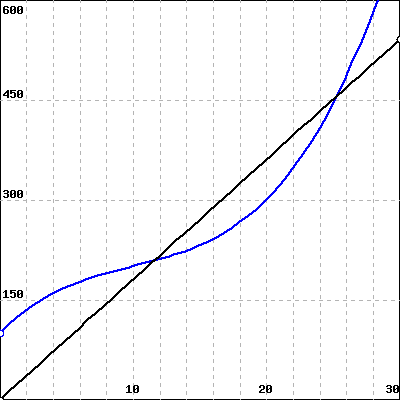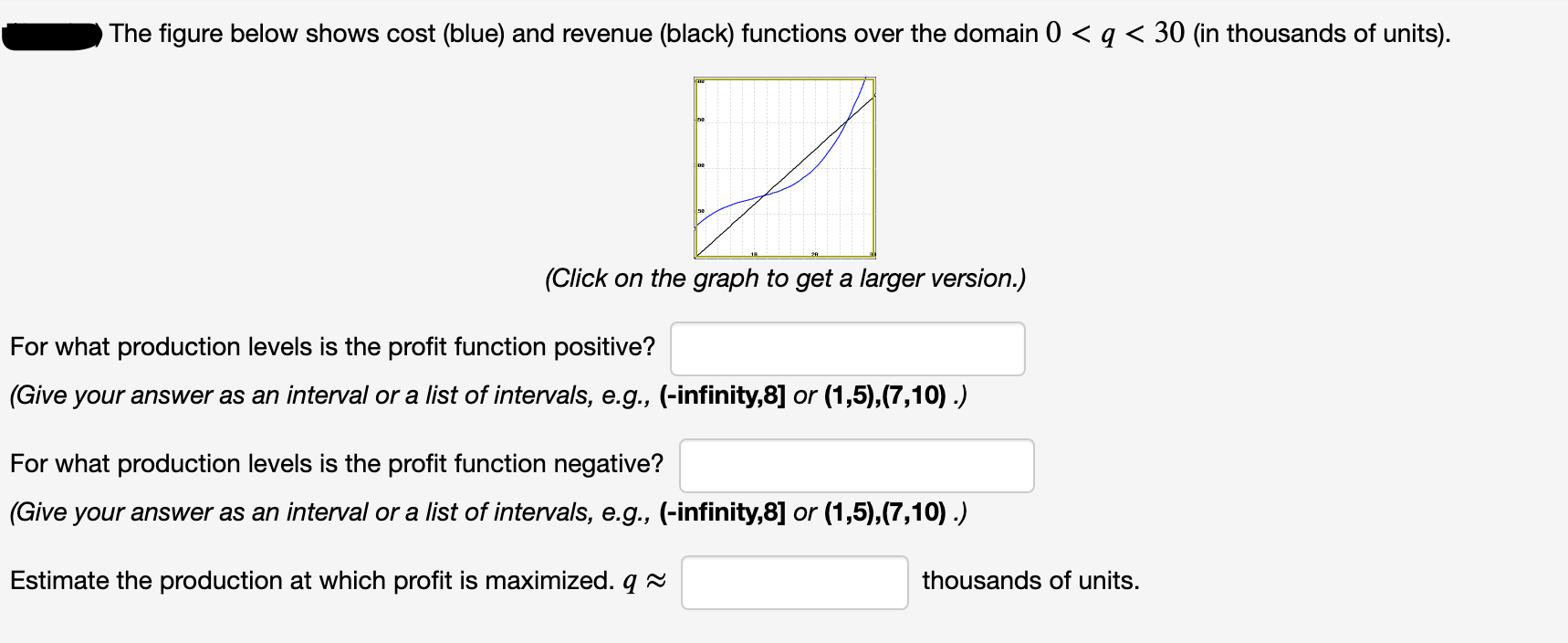# The figure below shows cost (blue) and revenue (black) functions over the domain 0 < q < 30 (in thousands of units). (Click on the graph to get a larger version.) For what production levels is the profit function positive? (Give your answer as an interval or a list of intervals, e.g., (-infinity,8] or (1,5),(7,10) .) For what production levels is the profit function negative? (Give your answer as an interval or a list of intervals, e.g., (-infinity,8] or (1,5),(7,10) .) Estimate the production at which profit is maximized. q 2 thousands of units.

Questionfullscreenhelp_outlineImage TranscriptioncloseThe figure below shows cost (blue) and revenue (black) functions over the domain 0 < q < 30 (in thousands of units). (Click on the graph to get a larger version.) For what production levels is the profit function positive? (Give your answer as an interval or a list of intervals, e.g., (-infinity,8] or (1,5),(7,10) .) For what production levels is the profit function negative? (Give your answer as an interval or a list of intervals, e.g., (-infinity,8] or (1,5),(7,10) .) Estimate the production at which profit is maximized. q 2 thousands of units. fullscreen

### Want to see this answer and more?

Experts are waiting 24/7 to provide step-by-step solutions in as fast as 30 minutes!*

*Response times vary by subject and question complexity. Median response time is 34 minutes and may be longer for new subjects.
Tagged in
MathCalculus

### Functions